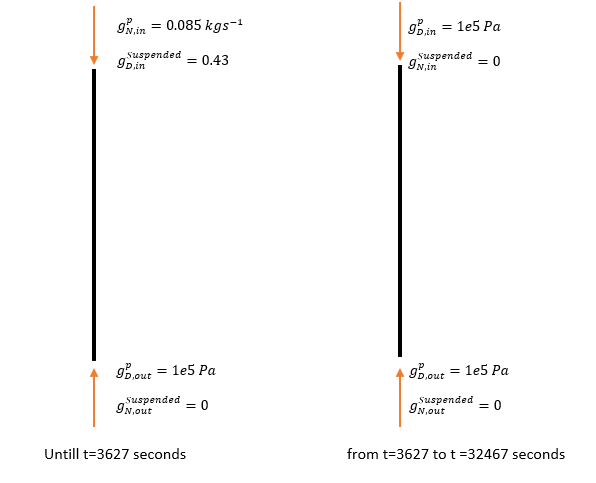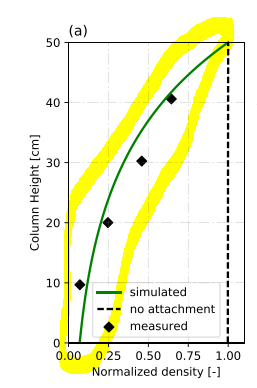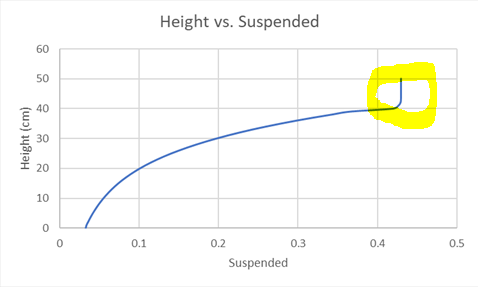# A Question (or problem) Regarding the Boundary Values

Hi,

I have a reactant transport model which includes biomass injection. The model has two phases, injection of biomass (defined as “Suspended” in the model) and retention of biomass. Initially, there is an injection of biomass from t=0 to t=3627 seconds. Then there is an 8 hr retention time, in which there is no injection or mass flux between time t=3628 to t=32467 second. Here is a simple sketch of described phases with boundary conditions. Initially, suspended concentration is zero, and the pressure is 1e5 Pa.Injected biomass reduces due to attachment and decay of the bacteria. The rate is kattMOL(“Suspended”)+kdMOL(“Suspended”), in which katt and kd are constants for attachment and decay. When I run the model, I see that the suspended concentration is not 0.43 till 3627 seconds at the top boundary. For example, at time t=900, 1800, it is 0.407715. At t=3600, there is an accumulation of suspended concentration, and from x=47 cm to x=50 cm, the concentration is 0.43, which I do not expect.
I expect a similar behavior shown in the figure below:But instead, I got this type of distribution at t=3600 s:Is there something wrong with my boundary conditions? I used DirichletWithinTime and Neumann boundary conditions together. But I don’t know what the problem is. I am attaching my file below.

BIOMASS.zip (301.7 KB)

Hi Emre,

Best regards,
Renchao

Hi Renchao,

I assigned a Dirichlet boundary condition to “Suspended” concentration (a value of 0.43) between time intervals of 0 and 3627 seconds at the top of the column (50 cm). But when I run the simulation, the suspended concentration at 50 cm is not 0.43 at t=900 and 1800 seconds. Why is that? Shouldn’t it be constant (0.43) between from t=0 to t=3627 s?

Another issue is why there is an accumulation at the top?I think attachment and decay rates are high enough to prevent this accumulation.

Thank you.

Hi Emre,

Strictly speaking, the solute concentration at the boundary where a Dirichlet boundary condition is assigned shall be absolutely as the same as the specified value. In your case, the concentration of the substrate you called “suspended” at the top boundary shall be 0.43 mol/m3. As you observed, the suspended concentration at that point virtually doesn’t equal to the value you assigned. This numerical artefact prevails in solving reactive transport problems, resulting from the adoption of the operator splitting algorithm under which the transport equation is solved along with the boundary conditions over the computational domain followed by solving the reaction equation. To my knowledge, the common practice is that the solution of the reaction equation is free from the rigid constraint of the prescribed Dirichlet boundary conditions. To prevent the solution inaccuracy from such numerical artefact, it is suggested to adopt small time step size for the simulation. Once you reduces the time step size from 50s you have set to 1s, the suspended concentration will be closer to the value of 0.43 mol/m3.

In my opinion, there remain a lot of work being done in the algorithm level to enhance the solution accuracy.

P.S.:
Please think about the boundary conditions you assigned for the substrate suspended. It looks weird to me that Dirichlet and Neumann boundary conditions are simultaneously assigned at the same boundary. I suggest you to consider using Dirichlet boundary condition incorporating the parameter type `CurveScaled` instead. I think it would be helpful to have a look at the benchmark OGS: ConTracer_1d.prj

Best regards,
Renchao

Renchao Hi,

When I adjust the time step as 1s, the phreeqc calculations do not converge, but 2 seconds works well.

Thanks for the curveScaled parameter suggestion, I am now working on that.

I’ve got a question regarding the specific body force. Should I insert -1 or -9.81? In some of the project files, it is -1; in some of them, it is -9.81. Should we insert the direction or quantity of the gravitational acceleration?

Hi Emre,
use -9.81 as a value. Depending on the problem’s dimensions it could be

``````<specific_body_force>0 -9.81</specific_body_force> <!-- for 2D -->
``````

or

``````<specific_body_force>0 0 -9.81</specific_body_force> <!-- for 3D -->
``````

Hi Dimitri,

Thank you for the information.

Best regards,
Emre

Hi Emre,